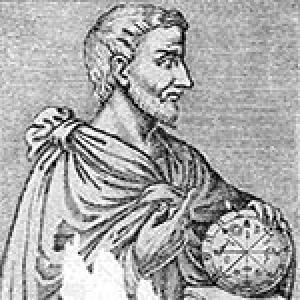## Mathematics problemDo you know the difference between a mystery and an enigma puzzle? An enigma puzzle is known to have a solution, but with a mystery you don’t know if there is solution.

Do you agree with this? 5 * 3 / 10 = ( 5 * 3 ) / 10 = 5 * ( 3 / 10 ) = 1.5

If you don’t, you should agree because it is a mathematical fact.

Now let’s do some experiments.

Try running the following code:

``````DATA val TYPE i.
val = ( 5 * 3 ) / 10.
WRITE val.``````

The answer on the screen is 2. Why is that? Simple. The variable is the integer type, and therefore it rounds off its value. If you change the variable type from I (integer) to F (float) you get 1.5 on the screen, thankfully.

Now try running the following code:

``````DATA val TYPE i.
val = 5 * ( 3 / 10 ).
WRITE val.``````

The answer on the screen is 0. Are we facing a mystery? Or is it just an enigma puzzle?

I have heard of people who have begun believing in God for less!

What is going on here can be easily explained. In ABAP, the results of intermediate calculations - in this case the division 3/10 - are also stored as a whole number variable. In other words: 3/10=0.333, which is rounded off to the nearest whole number, 0 in this case. Then 5*0=0. In the same way, if the variable in the second case is F (float), the answer shown is 1.5.

There you have it. The enigma puzzle is solved. Thank goodness, there was no mystery after all!

Thanks to Miguel Jorge for reminding me of this strange occurrence that is worthy of being the subject of an X-Files episode.

Greetings from Abapinho.